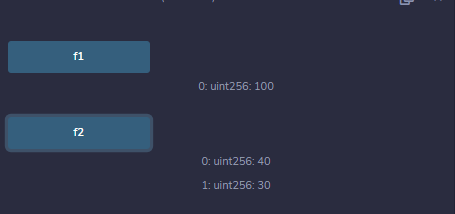# Solidity – Encapsulation

• Last Updated : 11 May, 2022

Encapsulation is fundamental and one of the most important concepts of object-oriented programming languages. It refers to the mechanism of manipulation of the scope of variables, i.e. it restricts the access of the variable outside the scope. It allows enough constraint access to a method for taking action on it. Scope of the objects can have four types of access:

1. Public: These objects can be accessed internally and externally as well as via messages also. Public elements can be inherited by external methods. A getter function is generated automatically when a public variable is created.
2. Internal: As the name suggests, Internal objects can be accessed by internal methods or by derived methods but cannot be accessed by external. Only a base contract and a derived contract has its access.
3. Private: Private objects can only be accessed internally from the current contract instances. They cannot be accessed by derived methods also.
4. External: These objects can be accessed externally but not internally i.e. current contract instances cannot access it. They cannot be inherited.

Example: In the below example, the contract parent is inherited by the contract child to demonstrate different scopes of the object discussed above.

## Solidity

 `// Solidity program to ``// demonstrate Encapsulation``pragma solidity ^0.5.0;`` ` `// Creating a contract``contract` `parent {`` ` `   ``// Declaring public ``   ``// state variable``   ``uint ``public` `num = 30;`` ` `   ``// Declaring internal ``   ``// state variable``   ``uint internal internal_num= 10;``    ` `   ``// Defining external function to ``   ``// demonstrate access of ``   ``// internal state variable``   ``function ``sqrt``() external returns (``     ``uint) {``      ``internal_num = internal_num ** 2; ``      ``return` `internal_num;``   ``}``}`` ` `// Defining calling contract``contract` `Caller {`` ` `   ``// Creating a child ``   ``// contract object``   ``child c = ``new` `child();`` ` `   ``// Defining public function ``   ``// to demonstrate access``   ``// to external function sqrt``   ``function f() ``public`  `returns (``     ``uint) {``      ``return` `c.``sqrt``(); ``   ``}`` ` `   ``// Defining function to ``   ``// demonstrate access to ``   ``// public functions increment() ``   ``// and add()``   ``function f2() ``public` `returns(``     ``uint, uint){``       ``return` `(c.increment(), c.add()); ``   ``}``}`` ` `// Defining child contract ``// inheriting parent contract``contract` `child is parent {`` ` `   ``// Defining public function ``   ``// to demonstrate access to ``   ``// public state variable num ``   ``function increment(``   ``) ``public` `payable returns (uint) {``      ``num = num + 20; ``      ``return` `num;``   ``}`` ` `   ``// Defining public function ``   ``// to demonstrate access``   ``// to local variable a, b, and sum``   ``function add() ``public` `view returns(``     ``uint){``      ``uint a = 10; ``      ``uint b = 20;``      ``uint sum = a + b;``      ``return` `sum;``   ``}``    ` `}`

Output:My Personal Notes arrow_drop_up